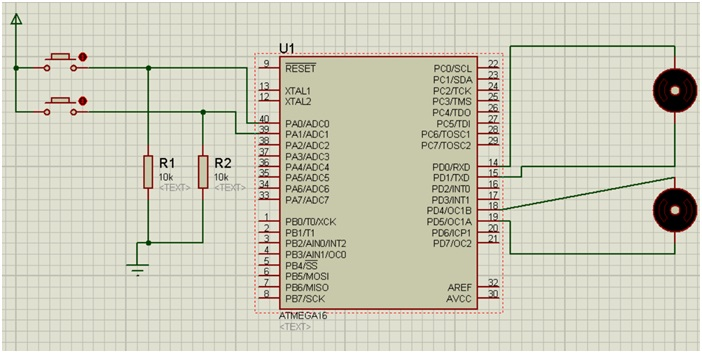# AVR | Control the revolution of Simple DC motor

In this article, we will learn how to use Push buttons to control the revolution of Simple DC motors? In this we will use two DC motors and two Push buttons; this would be easy for us to understand.
Submitted by Suryaveer Singh, on June 23, 2018

The simple concept that will be used here is similar like the cases that come in probability when two coins are tossed simultaneously, there our sample space would be HH, HT, TH, and TT.

Now in the case of DC motors consider 1 when our motor runs, and 0 if the motor doesn’t run. So here our sample space would be 11, 10, 01, and 00. Here all the four cases that we have to implement in our program that we will create.

## Program to control revolution of simple DC motor

```#include <avr/io.h>

int main(void)
{

DDRA=0x00;
DDRD=0x33;

while(1)
{
if((PINA&0x03)==0x03)
{
PORTD=0x11;
}
else if((PINA&0x03)==0x02)
{
PORTD=0x01;
}
else if((PINA&0x03)==0x01)
{
PORTD=0x10;
}
else if((PINA&0x03)==0x00)
{
PORTD=0x00;
}
}
}
```

Explanation:

• Here, DDRA=0x00 represents the inputs that are to be given by both the buttons which are connected in the PORT A.
• DDRD=0x03 represents the terminals in which our Motors are connected. Motor 1 is connected in terminal PD0 and PD1 while our motor 2 is connected in PD4 and PD5.
• ```PD7	PD6	PD5	PD4	PD3	PD2	PD1	PD1
0	0	1	1	0	0	1	1
```
• In the bottom line 0011 means 3 in hexadecimal so it is written as DDRD=0x33
• Inside the while loop we will use if/else statements to write all our four conditions 11, 10, 01, 00.
• The first if statement states our condition 11, this is the condition when our both the buttons will be pressed and our both the motor will rotate here.
• Inside the, if statement PINA & 0x03 is basically a statement which tells us the condition of number of button Pressed.
• If both the buttons are pressed (0x03) then PORTD==0x11 i.e. both the motors will run simultaneously.
• If only second button is pressed (0x02) then PORTD==0x01 i.e. only first motor will start to rotate.
• If only first button is pressed (0x01) then PORTD=0x10 i.e. only second motor will start to run.
• That last condition states that if no button is pressed (0x00) i.e. PORTD==0x00, here no motor will run.

Simulation:Explanation:

• Device Required:
• Two Resistors of 10k ohm
• Ground Terminal
• Power Terminal
• Two push buttons
• Atmega16
• Two simple Active DC motors (double click on them and change the value from 12v to 3v)
• Arrange the components as shown in the figure above.
• Change the motor voltage value as mentioned above.
• Debug the HEX file in the ATmega16.
• Click on the play button and your simulation will start.

TRICK:

If after creating 2-3 programs if you have noticed that you face problem is writing code for the pins , than I would suggest you to first create the design of the simulation in the PROTEUS software then with reference to that write the Pin configurations in Program.

What's New

Top Interview Coding Problems/Challenges!

Languages: » C » C++ » C++ STL » Java » Data Structure » C#.Net » Android » Kotlin » SQL
Web Technologies: » PHP » Python » JavaScript » CSS » Ajax » Node.js » Web programming/HTML
Solved programs: » C » C++ » DS » Java » C#
Aptitude que. & ans.: » C » C++ » Java » DBMS
Interview que. & ans.: » C » Embedded C » Java » SEO » HR
CS Subjects: » CS Basics » O.S. » Networks » DBMS » Embedded Systems » Cloud Computing
» Machine learning » CS Organizations » Linux » DOS
More: » Articles » Puzzles » News/Updates